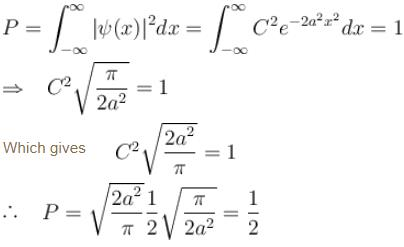Courses

# Postulates Of Quantum Mechanics NAT

## 10 Questions MCQ Test Topic wise Tests for IIT JAM Physics | Postulates Of Quantum Mechanics NAT

Description
This mock test of Postulates Of Quantum Mechanics NAT for IIT JAM helps you for every IIT JAM entrance exam. This contains 10 Multiple Choice Questions for IIT JAM Postulates Of Quantum Mechanics NAT (mcq) to study with solutions a complete question bank. The solved questions answers in this Postulates Of Quantum Mechanics NAT quiz give you a good mix of easy questions and tough questions. IIT JAM students definitely take this Postulates Of Quantum Mechanics NAT exercise for a better result in the exam. You can find other Postulates Of Quantum Mechanics NAT extra questions, long questions & short questions for IIT JAM on EduRev as well by searching above.
*Answer can only contain numeric values
QUESTION: 1

### The wave function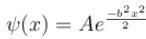where A and B are real constant, is a normalised eigenfunction of the Schrödinger equation for a particle of mass M and energy E in a one dimensional potential V(x) such that V(x) = 0 at x = 0. Find the value for V in units of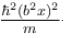Solution:

Applying time independent Schrödinger equation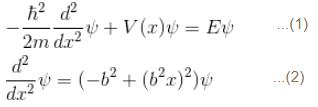Substituting (2) in (1)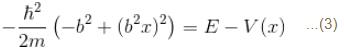Putting V(x = 0) = 0  in (3)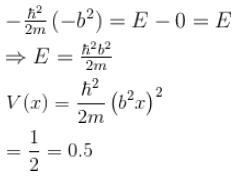*Answer can only contain numeric values
QUESTION: 2

### In the spectrum of hydrogen, what is the ratio of the longest wavelength in the Lymann series to the longest is Balmer series ?

Solution:

Energy emitted due to transition of electron -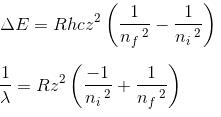Wherin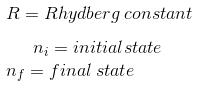Longest wavelength in balmer series is corresponding to transition between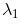Lets say it is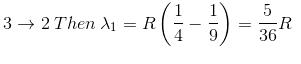Longest wavelength in lymen series is corresponding to transition between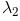2 ---> 1

Lets say it is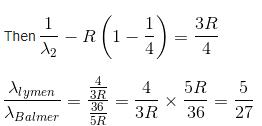=0.1852

*Answer can only contain numeric values
QUESTION: 3

### In a photoelectric effect set up two metals with work function  φ1 and φ2 are used. For the same value of incident frequency, the stopping potential for metal 1 is twice that for metal 2. If the incident energy is 5eV and the φ1 = 1.5eV.  Find  φ2. (in eV)

Solution:

Solution=

eVs1=hv- Φ1

eVs2=hv- Φ2

eVs1= eVs2

eVs1/eVs2 = hv- Φ2/ hv- Φ1=1/2

2hv-2Φ= 1hv- Φ1

= hv+ Φ1

= 5+1.5

= 6.5eV

Φ= 3.25eV

The correct answer would be 3.25eV

*Answer can only contain numeric values
QUESTION: 4

A particle is in an infinite square well potential with walls at x = 0 and x = L. If the particle is in the state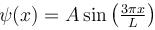where A is a constant, what is the probability that the particle is between x = L/3 and  x = 2L/3 upto 2 decimal places.

Solution: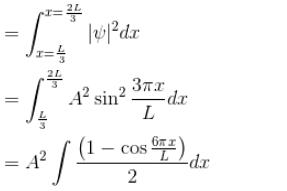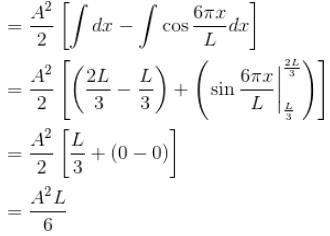Now A2 = 2/L = from normalization of ψ
∴    Probability = 1/3 = 0.33

*Answer can only contain numeric values
QUESTION: 5

The figure shows one of the possible energy eigenfunction ψ(x) for a particle bouncing freely back and forth along the x-axis between impenetrable walls located at x ± a The potential energy equals zero for |x| < a. If the energy of the particle is 2eV when it is in the quantum state associated with its eigen function, what is its energy when it is in the quantum state of lowest energy (in eV)?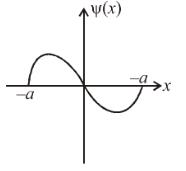Solution:

The number of nodes in a wavefunction determine the energy level. In this case there is just one node. Thus, this is E2. The lowest would be E1.
En = (const)n2
Since E2 = 2 = (constant) 4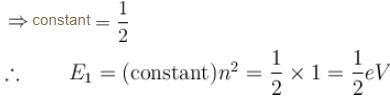*Answer can only contain numeric values
QUESTION: 6

What is the probability of finding the particle in classically forbidden region in ground state of simple harmonic oscillator

Solution:

*Answer can only contain numeric values
QUESTION: 7

An electron in a metal encounters a barrier layer of height 6eV and thickness 0.5 nm. If the electron energy of 5eV, what is the probability of tunneling through the barrier?

Solution:

We know,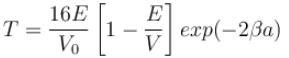With  E = 5eV  and  V0 = 16eV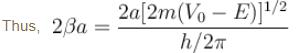With  a = 0.5 × 10–9 m,  m = 9.1 × 10–31 kg,  V0 = 6eV,  E = 5V   and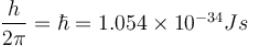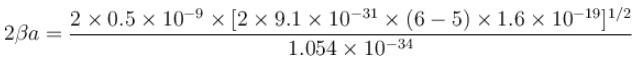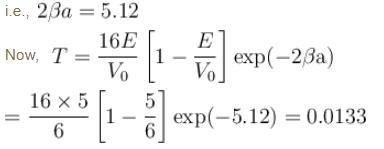Hence the percentage of probability of tunneling is 1.33.

*Answer can only contain numeric values
QUESTION: 8

The energy of an electron moving in one dimension in an infinitely high potential box of width 1Å.

Solution:

The eigenvalue of energy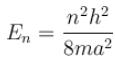when the particle is in the least energy state (n = 1), the energy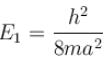with h = 6.62 × 10–34 Jsm = 9.1 × 10–31 kg and a = 1 × 10–10 m

After calculating,

The correct answer is: 37.62 eV

*Answer can only contain numeric values
QUESTION: 9

The solution of the schrödinger equation for the ground state of hydrogen is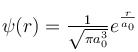where a0 is the Bohr radius and r is the distance from the origin. The most probable value of r  in units of a0.

Solution:

For a hydrogen atom,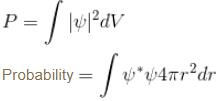Now, for most probable r. We need to minimize the probability w.r.t. r.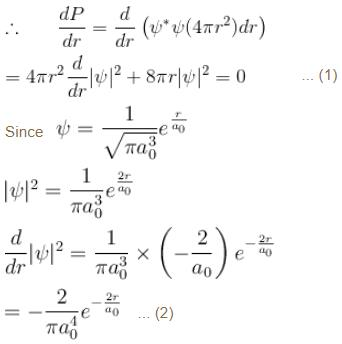Putting (2) in (1)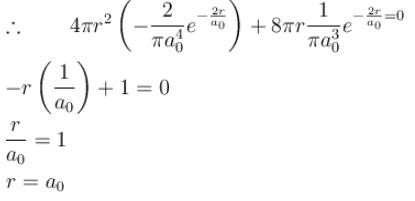*Answer can only contain numeric values
QUESTION: 10

The wave function of a particle is given by ψ(x) = Ce-ax,  -∞ < x < + ∞ where C and a are the some constants. The probability of finding the particle in the region  0 < x < ∞

Solution:

The probability of finding the particle in the region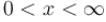isApplying normalising condition i.e.,